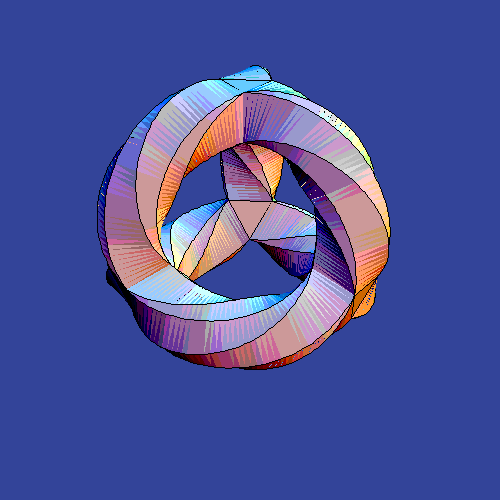Friday, June 21, 2019

McAllister et al.: weak gravity makes some low-volume cycles mandatory

Out of the 14 new primarily hep-th papers today, about 8 (a majority) may be counted as "string theory" which is great. Even more impressively, 4 papers are about Calabi-Yau manifolds. Let me look at the first one – which was posted at 18:00:00 UTC, guaranteeing the first place. These guys may have mastered the correct calculation of the delay after you press the enter key and after the packets get to the arXiv.A counting problem involving the Calabi-Yau manifolds is one of the most popular examples of the deep implications that string theory has for the world of mathematics.

A real-six-dimensional manifold of a particular topology, the quintic (or the quintic hypersurface), has some non-contractible lower-dimensional manifolds in it. Some of them are (complex) "lines" in some algebraic sense. Their number has been known to be 2875 for quite some time. The more complex submanifolds, the "conics", are more numerous. Mathematicians have only known that their number was 609,250 since 1986.

To count the higher-degree curves seemed like an impossibly difficult problem for the mathematicians. Suddenly, string theorists arrived and claimed that the Calabi-Yau manifold above was a mirror dual to another – string theory on both manifolds may be exactly physically equivalent – and physicists were therefore able to easily compute as many numbers in the sequence as they wanted, thus proving that string theorists are better mathematicians than the mathematicians.

As all TRF readers surely know by heart, the next two entries are 317206375 and 242467530000.

Mirror symmetry is surely deep in this sense. I've been uncertain whether the Weak Gravity Conjecture is comparably deep. It's just some lame and seemingly obvious claim about the strength of the forces, right? Well, Kevin McAllister and his family (Cornell+Northeastern – but ancestrally, Stanford's settlements on the East Coast) elaborated on a clever way to show that the Weak Gravity Conjecture has deep implications for geometry which are somewhat analogous to the counting problem for the Calabi-Yau manifolds:
Minimal Surfaces and Weak Gravity
OK, the first name isn't Kevin but I wanted to increase the traffic to arXiv.org. The main reason why the seemingly dull Weak Gravity Conjecture has implications for the higher-dimensional geometry is that the electromagnetic forces may be obtained from rather complex higher-dimensional constructions within string theory.

They discuss Euclidean D3-brane instantons on 4-cycles of Calabi-Yau three-folds in type IIB string theory. I feel somewhat anxious about the instanton form of the Weak Gravity Conjecture. Liam and others, wouldn't be it at least equally logical to talk about type IIA string theory and D4-branes that wrap four-cycles to produce simple charged and massive particles? That's really the normal way to formulate the Weak Gravity Conjecture.

OK, I will use the D4-brane language. If you wrap the D4-brane on a supersymmetric 4-cycle, the attractive gravitational force will be the same – up to an undetermined sign – as the $$U(1)$$ repulsive "electric" force where the $$U(1)$$ field comes from a contraction of a Ramond-Ramond field over the four-cycle. BPS objects clearly pass the Weak Gravity Conjecture marginally by saturating the inequality.

But what about the non-supersymmetric cycles? Elements of the homology that are some general linear combination of holomorphic and antiholomorphic cycles? The mass of a D4-brane wrapped on this general cycle is equal to the tension of the D4-brane times the volume of the 4-cycle. The cycles also produce the electric forces, as I said, and the magnitude of the charge – normalized to make the electric force natural – is given by the Kähler potential,$\sqrt{\Sigma_i (K^{-1})^{ij} \Sigma_j }.$ The force obeys ${\rm Re} \,S_\Sigma \leq c ||\Sigma||$ where $$c$$ is of order one and they actually determine it to be $$c=\sqrt{3/4}$$ for their particular class of examples. I just want to make sure that you don't think that this $$c$$ makes the inequality vacuous.

OK, the gravitational force must be weaker than the electric one for this non-supersymmetric cycle which means that the minimum-volume submanifold in the non-supersymmetric cohomology class must be smaller than something. They decide that their final inequality is${\mathfrak r}_\Sigma \geq {\mathfrak r}_\Sigma^{\rm min} := \frac{2\pi}{c} \frac{{\rm Vol} (\Sigma_\cup)}{||\Sigma||} - 1. \tag{2.11}$ This may become highly nontrivial because the lattice of possible charges may become sparse or very sparse. Even though you have a small amount of freedom to pick the representative, a cycle of a surprisingly low, "sub-Pythagorean" volume is guaranteed to exist.

I think their claim is some generalization of a schoolkid's statement that there exists a connection between points $$(x,0)$$ and $$(0,y)$$ whose length is at most $$\sqrt{x^2+y^2}$$. Except that their statement replaces the linear, flat line intervals $$x$$ and $$y$$ with some curved submanifolds of a 6-dimensional manifold.

An alternative outcome could be, of course, that the Weak Gravity Conjecture is wrong. You could disprove the Weak Gravity Conjecture in a new way if you found a Calabi-Yau manifold and proved that the low-volume four-cycle whose existence they have derived doesn't exist. You must be warned: you're rather unlikely to succeed because such a result would also mean, basically, that our paper is wrong and it is a tall order. ;-) More seriously, there exist conceptual arguments – arguments that come from several lines of reasoning and end up with the same conclusion – that derive the conclusion from some solidly motivated statements about black holes, forces, quantum gravity, and string theory.